Next: C..2.6 Putting It All Up: C..2 Lighting Equations Previous: C..2.4 Diffuse Term   Contents

### C..2.5 Specular Term

The specular term also depends on whether light falls directly on the vertex. If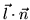is less than or equal to zero, there is no specular component at the vertex. (If it's less than zero, the light is on the wrong side of the surface.) If there's a specular component, it depends on the following:

• The unit normal vector at the vertex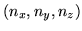.
• The sum of the two unit vectors that point between (1) the vertex and the light position and (2) the vertex and the viewpoint (assuming that GL_ LIGHT_MODEL_LOCAL_VIEWER is true; if it's not true, the vector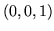is used as the second vector in the sum). This vector sum is normalized (by dividing each component by the magnitude of the vector) to yield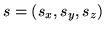.
• The specular exponent (GL_ SHININESS).
• The specular color of the light (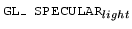).
• The specular property of the material (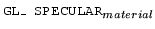).

Using these definitions, here's how OpenGL calculates the specular term: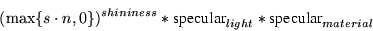However, if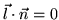, the specular term is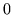.Next: C..2.6 Putting It All Up: C..2 Lighting Equations Previous: C..2.4 Diffuse Term   Contents
2001-01-10